## Boost converter - Continuous conduction mode (CCM)

##### Introduction

The objective of this experiment is to study the characteristics of a boost converter. The circuit will be operated under continuous current mode (CCM) and open loop conditions (no feedback).

##### Theoretical background

A boost converter is shown below, with the transistor and the diode making up the bi-positional switch of the power pole.Turning on the transistor increases the inductor current, thereby the energy stored in the inductor and when the transistor is turned off, the inductor current flows into the output capacitor through the diode, thereby transfering the incremental energy to the capacitor. For a given transistor switching function waveform q(t), shown below, with a switch duty ratio d in steady state, the waveform of the voltage vA at the current port follows q(t) as shown.When the switch is ON, i.e., q(t) = 1, the voltage across the inductor is Vin. When the switch is OFF, i.e., q(t) = 0, the voltage across the inductor is Vin - Vo. Since the average voltage across the inductor under steady state is zero, from the vL plot in the above image

d × Ts × Vin + (1 - d) × Ts × -(Vo - Vin) = 0

⇒ Vo = Vin1 - d(1)

The inductor current iL comprises of the average inductor current output IL which equals the output current Iin, and the ripple current iL,ripple which ideally flows into the capacitor.

iL(t) = IL (= Iin) + iL,ripple(t)(2)

The diode current idiode is same as the inductor current when the switch is OFF and 0 when the switch is ON. This current is the sum of the output current Io,

Io = VoR(3)

and the capacitor current ic.

For an ideal boost converter the input power equals the output power

VinIin = VoIo(4)

Using Eqn. 1 through Eqn. 4

IL = Iin = VoVinIo = 11 - dVoR(5)

The inductor ripple current component is dicated by the inductor voltage since iL(t) = 1L∫vL(t) ⋅ dt. Thus, over a switching cycle, the peak-peak ripple current is given by

ΔiL = VinL × d × Ts = Vo - VinL × (1 - d) × Ts(6)

##### Preparing the Workbench model
1. Copy the folder where pre-built example project for this experiment is present, usually in C:\Program Files (x86)\Sciamble\WorkBench v1\Examples\CUSPLab\BasicPowerElectronics\Experiment5 and paste it in a location where the user has permission to edit and save files, like the Desktop folder.

2. Launch Workbench application.

3. Pin (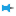) the Explorer dock on the right and the Toolbox dock on the left.

4. Click the second icon within the Explorer dock,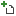to open the project.

5. Navigate to the folder where the pre-built example project was pasted in Step 1. Double click the BoostConverter.project node within that folder to open the project.

6. Click the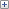icon to explore the files within the project. Double click the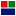Boost node in the Explorer dock to display the model file.

7. Ensure that the switching frequency is set to 100 kHz. To do this, double click on the project node,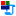BoostConverter, in the Explorer. Go to the next page by clicking the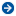in the property dock or choosing Device configuration from the drop-down menu. Check that the Frequency within PWM Configuration is set to 100000.

8. Ensure that the PWM channel is set to the Si power pole. To do this, open the property of the tool labelled Si PWM in the model by double clicking on it. Check that the Channel is set to 2.

In buck converter Channel was set to 1, to use the top switch of a two-switch power-pole. In boost converter Channel is set to 2, to use the bottom switch of a two-switch power-pole.

##### Preparing the setup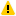Warning

Before proceeding, ensure that the isolated power supply is powered down and that the USB cable is disconnected.

1. Magnetics card connection: Unscrew any existing magnetics card and replace it with the Buck/Boost/Buck-boost magnetics card. Prior to connecting the card, ensure that the jumper on the bottom of the magnetics card is removed. Make sure that all the 6-pins of the magnetics card are making solid contact with the power-pole board.Warning

Never leave the magnetics card unscrewed. If contact is lost while the converter is running, it has the potential to cause very high voltage due to interruption of inductor current, potentially damaging the converter, or worse, could lead to a safety hazard.

2. Rheostat setting: Set the slider such that the resistance across the two closest rheostat terminals is 40 Ω.

3. Power connections:

Boost converter connections are the same as that of the buck converter where the input and output terminals are swapped.

1. Connect the Vin terminals to the rheostat load:

1. Rheostat rail: Vin+ (Red)

2. `Rheostat's other terminal that is closest to the rail: ● Vin− (Black)`

2. Connect the Vo terminals to the isolated power supply:

1. DC +ve: Vo+ (Red)

2. `DC −ve: ● Vo− (Black)`

3. Connect the power supply ground to the ground terminal on the board:

Ground: Earth (Green)

4. DSO connections:

1. Connect DSO channel 1 probe to Io. Set the following options, if they are supported by the DSO:

1. Set the probe to 1x.

2. Set the measurement type as Current.

3. Set the scaling factor to 5x.

4. Set the offset at 1.5 V or 7.5 A.

5. Set termination to 1 MHz.

6. Set the channel as default/non-inverted.

2. Connect DSO channel 2 probe to SWA. Set the following options, if they are supported by the DSO:

1. Set the probe to 1x.

2. Set the measurement type as Voltage.

3. Set the scaling factor to 1x.

4. Set the offset at 0 V.

5. Set termination to 1 MHz.

6. Set the channel as default/non-inverted.

3. Connect DSO channel 3 probe to Iin. Use the same setting as that of Channel 1.

4. Connect DSO channel 4 probe to Vin. Use the same setting as that of Channel 2.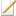Note

Since the input and output power terminals are swapped, Channel 1 which is connected to Io, actually measures the input/inductor current.Channel 3 which is connected to Iin, actually measures the diode current and Channel 4 which is connected to Vin, actually measures the output voltage.

5. Jumper settings:

1. Insert the Vdc jumper.

2. Insert the GaN/Si jumper to the Si side.

3. Insert the GaN switch's external diode jumper, labelled "Diode".

4. The jumper on the bottom of the magnetics card was removed in Step 1, while inserting the card.

6. Connect the USB cable to the power-pole board and the computer.

7. DC power supply settings:

1. Make sure that the DC power supply is fully turned down to 0 V prior to turning on the supply.

2. Turn on the power supply and gradually ramp up the voltage from 0 V to 15 V.

3. If the option is available, set the power supply current limit at 4.5 A.

The final wiring should look similar to this: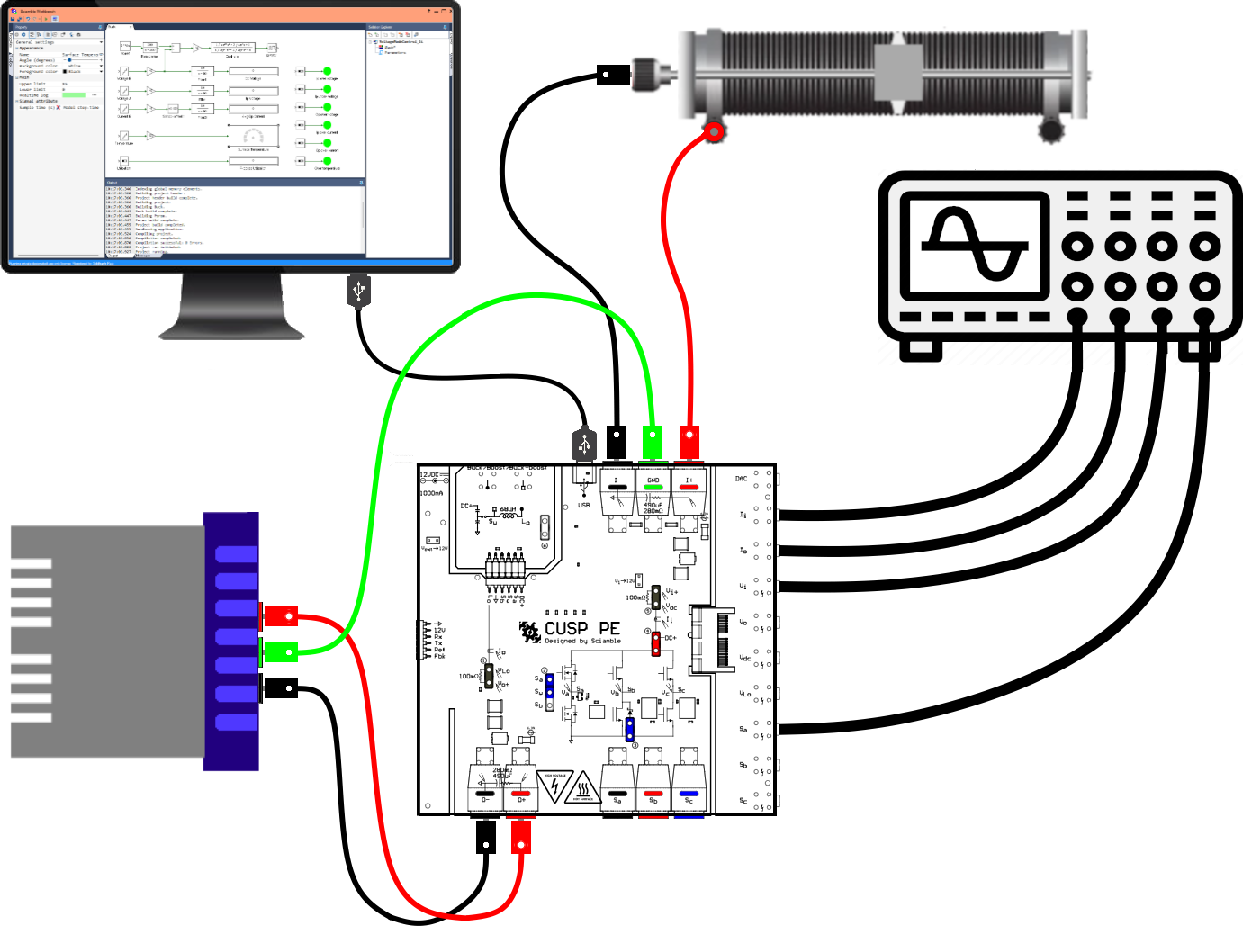##### Real-time open loop control of boost converter - varying duty cycle
Running the setup:
1. Click on the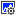icon in the top dock of Workbench to transition from the simulation mode to the real-time mode.

2. Boost converter, unlike the buck converter, has non-zero current at zero duty cycle. To compensate for the current sensor offset, the reading must be taken at zero current. Since that is not feasible in the boost converter configuration, use the values from the previous Buck converter - Continuous conduction mode (CCM) lab experiment.

Open Sensor offset tool's property by double clicking on it and set the Offset value to the negative of the measured Avg Ip Current in the previous step.

Open Sensor offset1 tool's property and set the Offset value to the negative of the measured Avg Op Current in the previous step.

3. Click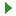to run the control algorithm in real-time.

4. Gradually increment the duty cycle from 0 to 0.5 in steps of 0.05. If a fault occurs, stop the model by clicking on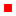button.

5. Make the following measurements:

1. Make a note of the voltage and current values displayed on Workbench, for each duty cycle step.

2. Observe the DSO waveforms and make a copy of the voltage across the switch (channel 2), the output voltage (channel 4), the inductor/input current (channel 1), and the diode current (channel 3) for duty cycle of 0.1, 0.2, 0.3, and 0.4. Adjust the time base to show anywhere between 4-10 switching cycles.

6. Click onto stop the model.

##### Real-time open loop control of boost converter - varying switching frequency
Running the setup:
1. Set the switching frequency to 100 kHz. To do this, double click on the project node,BoostConverter, in the Explorer. Go to the next page by clicking thein the property dock or choosing Device configuration from the drop-down menu. Change that the Frequency within PWM Configuration to 100000.

2. Clickto run the control algorithm in real-time.

3. If an undervoltage fault occurs, slightly increase the input voltage above 15 V but less than 16 V. Stop the model by clicking onand rerun it.

4. Gradually increment the duty cycle from 0 to 0.3. If a fault occurs, stop the model by clicking onbutton and re-run the model.

5. Make the following measurements:

1. Make a note of the voltage and current values displayed on Workbench, at 0.3 duty cycle.

2. Observe the DSO waveforms and make a copy of the voltage across the switch (channel 2), the output voltage (channel 4), the inductor/input current (channel 1), and the diode current (channel 3) at 0.3 duty cycle. Adjust the time base to show anywhere between 4-10 switching cycles.

Measure the peak-peak inductor ripple current. This will be used to compute the inductance value.

6. Click onto stop the model.

7. Repeat this section for PWM frequency of 80 kHz, 60 kHz, and 40 kHz. For these frequencies, it is sufficient to take measurement at just a single duty cycle of 0.3.

8. Turn OFF the power supply and disconnect the USB cable.

If required, repeat the same experiment using GaN power-pole instead of Si power-pole as demostrated in Switching characteristic of Si MOSFET/GaN FET and diode.

##### Lab report and reading assignment
1. Attach the DSO waveforms showing the voltage across the diode, the output voltage, the inductor current, and the input current for switching frequency of 100 kHz and duty cycles of 0.1, 0.2, 0.3, and 0.4.

2. Attach the DSO waveforms showing the voltage across the diode, the output voltage, the inductor current, and the input current for duty cycle of 0.3 and switching frequency of 40 kHz, 60 kHz, 80 kHz, and 100 kHz.

3. For switching frequency of 100 kHz and duty cycle varying from 0.1 to 0.5 in steps of 0.05, enter the measured values from the Workbench screen capture and calculate the following values:

dset Vin (V) Iin,avg (A) Pin (W) Vo (V) Io,avg (A) Pout (W) dact Efficiency (%)
`        `
`        `
`        `
`        `
`        `
`        `
`        `
`        `
`        `

where, dset is the duty cycle value set using Workbench and dact is the duty cycle (d) calculated using Eqn. 1.

Plot the efficieny as a function of dutyact.

Plot the Vo as a function of dutyset.

4. For duty cycle of 0.3 and switching frequency of 40 kHz, 60 kHz, 80 kHz, and 100 kHz, enter the recorded values from Workbench and calculate the following values:

fsw (kHz) Vin (V) Iin,avg (A) Pin (W) Vo (V) Io,avg (A) Pout (W) Efficiency (%)
`        `
`        `
`        `
`        `
`        `
`        `
`        `
`        `

Plot the efficieny as a function of fsw. Comment on the difference in efficiency with respect to switching frequency.

5. From the plot of inductor current at 100 kHz switching frequency, duty cycle of 0.3, and load resistance of 40 Ω, estimate the boost converter inductor value using Eqn. 6.

##### Reference
1. "Power Electronics, A First Course," Ned Mohan and Siddharth Raju, Wiley Publication.